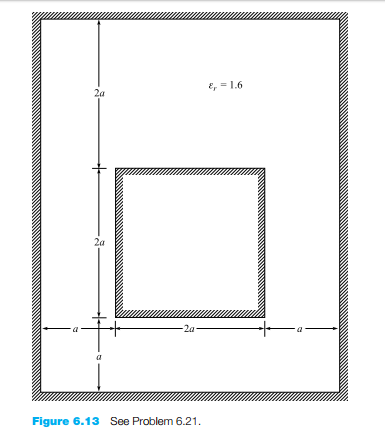### Create an Account

Home / Questions / A two wire transmission line consists of two parallel perfectly conducting cylinders each ...

# A two wire transmission line consists of two parallel perfectly conducting cylinders each having a radius of 02 mm separated by a center to center distance of 2 mm The medium surrounding the wires

A two-wire transmission line consists of two parallel perfectly conducting cylinders, each having a radius of 0.2 mm, separated by a center-to-center distance of 2 mm. The medium surrounding the wires has ϵr = 3 and σ = 1.5 mS/m. A 100-V battery is connected between the wires. (a) Calculate the magnitude of the charge per meter length on each wire. (b) Using the result of Problem 6.16, find the battery current.Jun 14 2020 View more View LessSubscribe To Get Solution